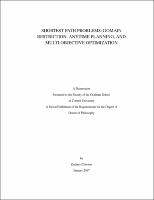## Shortest Path Problems: Domain Restriction, Anytime Planning, and Multi-objective Optimization##### Abstract
Optimal path problems arise in many applications and several efficient methods are widely used for solving them on the whole domain. However, practitioners are often only interested in the solution at one specific source point, i.e. the shortest path to the exit-set from a particular starting location. This thesis will focus on three separate, but related, problems of this form. We employ solution methods that discretize the computational domain and recover an approximate solution to the shortest path problem. These methods either solve the problem on a geometrically embedded graph or approximate the viscosity solution to a static Hamilton-Jacobi PDE. Such paths can be viewed as characteristics of static Hamilton-Jacobi equations, so we restrict the computations to a neighborhood of the characteristic. We explain how heuristic under/over-estimate functions can be used to obtain a {\em causal} domain restriction, significantly decreasing the computational work without sacrificing convergence under mesh refinement. The discussed techniques are inspired by an alternative version of the classical A* algorithm on graphs. We illustrate the advantages of our approach on continuous isotropic examples in 2D and 3D. We compare its efficiency and accuracy to previous domain restriction techniques and analyze the behavior of errors under the grid refinement. However, if the heuristic functions used are very inaccurate this can lead to A*-type methods providing little to no restriction. One solution is to scale-up the underestimate functions used so that they become more accurate on parts of the domain. However, this will cause the algorithm to recover suboptimal, albeit locally optimal, solutions. These algorithms quickly produce an initial suboptimal solution that is iteratively improved. This ensures early availability of a good suboptimal path before the completion of the search for a globally optimal path. We illustrate the algorithm on examples where previous A*-FMM algorithms are unable to provide significant savings due to the poor quality of the heuristics. Finally we present a related algorithm for finding optimal paths on graphs with respect to two criteria simultaneously. Our approach is based on augmenting the state space to keep track of the budget'' remaining to satisfy the constraints on secondary cost. The resulting augmented graph is acyclic and the primary cost can be then minimized by a simple upward sweep through budget levels. The efficiency and accuracy of our algorithm is tested on Probabilistic Roadmap graphs to minimize the distance of travel subject to a constraint on the overall threat exposure to enemy observers.
2017-01-30
##### Keywords
Partial differential equations; Robotic path-planning; Applied mathematics; Bi-objective optimization; Graph theory; Numerical analysis; Optimal control
##### Committee Member
Guckenheimer, John Mark
Bindel, David S.
##### Degree Discipline
Applied Mathematics
##### Degree Name
Ph. D., Applied Mathematics
##### Degree Level
Doctor of Philosophy